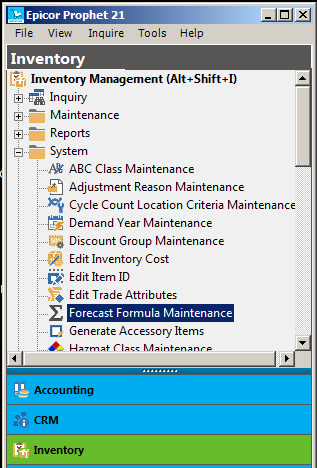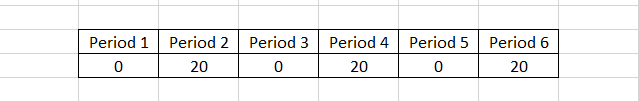# Prophet 21 Tutorial: Forecast Formula MaintenanceToday’s Prophet 21 tutorial is focused on how to edit and maintain Epicor P21 ERP’s formulas that are used for forecasts.  You may have seen earlier posts or other training docs that talk about “best fit formulas”.  This post is going to guide you through what that means, and how the formulas in P21 are used.

# What is a Best Fit Formula?

Most modern forecasting systems use some type of best fit formula model to base the forecast on.  In a nutshell, this means that past history is used to find a pattern of usage.

When Prophet 21 forecasts an item, the usage history is put into each of the forecast formulas defined for the appropriate item velocity.  If the item is seasonal, only the seasonal formulas are used.  The forecast error for each of those formulas is compared to assess which formula provided the lowest error rate.  This formula is then selected as the “best fit” for that item.  Every formula is built on the idea that each period (month) in the formula is assigned a certain weight.

When the best fit formula is found, it is then applied to that item until the next time a re-forecast is run.  The forecast can be started in the Demand Year Maintenance screen.  It can also be set up to run automatically.  My Github page provides a detailed, turn-key script you can run to create the automatic forecasting job.

# Prophet 21 Tutorial: Edit a Forecast Formula

Editing a forecast formula is not a difficult thing.  A little knowledge is required to get your custom tuned formula set up. Other that that it is pretty straightforward.  The challenge of editing a formula is deciding what to edit the formula to do.  Fortunately, Prophet 21 comes with several default formulas.  Additionally there are other resources on the web that can help you with understanding forecast formulas.  Regular readers here at FreeingERP know that I am a fan of Jon Schreibfeder’s approach to forecasts, and this topic is no exception.  This post on effectiveinventory.com is a great place to start.

The main thing to keep in mind when editing a P21 forecast formula is what I have come to call the “Rule of Ten”.  The easiest way I can explain this is that the average of every period in your formula must equal ten.  At least this is what I was advised by Epicor a couple years back.  It seems to hold true as best I can tell.

#### Rule of Ten Example

As an example, if your formula has a value in all 12 periods, then the total weight of all values must equal 120 (120 divided by 12 is 10).  Likewise if your formula has values in 6 out of the 12 periods, then the total weight of all 6 periods must be 60.  Once you have your head wrapped around this concept, the rest of the process is very easy.The above screen shot shows the Forecast Formula Maintenance window for moderate velocity items.  It is accessed on the menu through Inventory -> Inventory Management -> System -> Forecast Formula Maintenance.  From here, you can make tweaks to the formulas to suit your needs, just don’t forget about the “Rule of Ten”.   To edit a formula, just add a row, input your formula and save.  If you were tuning an existing formula, make sure you set the original version to deleted.  Formulas in Prophet 21 are applied based on the item’s velocity, so if you want to have a universal formula, make sure to set it up on each velocity tab.

Disclaimer: I highly stress that you test formulas in Play and/or in a spreadsheet before you update them in a live database.  As I said before, the challenge is not the editing, the challenge is in knowing how to make the edit in the first place.

### Prophet 21 Tutorial Tip: On Again Off Again Sales

If you are like most distributors, you have certain items that seem to be hit and miss.  Maybe a dominant customer is buying every other month, making your forecasts very inaccurate.  This also makes buying that item very hard.  The screenshot below is a tip on how to build an alternate period forecast formula to help you deal with these kinds of items.This formula assigns weights based on having sales every other period.  You can assign weights however you like to get this dialed in, but this type of formula can help you deal with a very common problem for many distributors.

# Key Takeaways and Wrap Up

I hope you found this Prophet 21 tutorial on forecast formulas useful and informative.  Again, remember to test in a play database before deploying any changes to a live environment.  The main points from this article to keep in mind are:

1. Don’t forget the Rule of Ten.  The average value of all period weights must equal 10
2. Do your research before deciding to edit a formula.  Formula tuning can be very helpful if you know how to do it.
3. Prophet 21 uses best fit forecasting.  An item is bounced against each formula, and the one with the lowest error rate is selected.
4. If you want to use formula edits immediately, run a re-forecast in Demand Year Maintenance.

To get more Prophet 21 tutorial posts from FreeingERP, check out our Prophet 21 category archives here.

Posted in Inventory Management, Prophet 21 and tagged , , , .